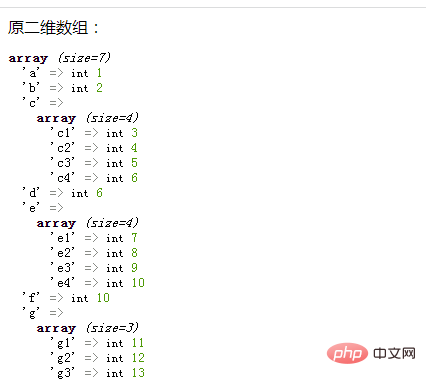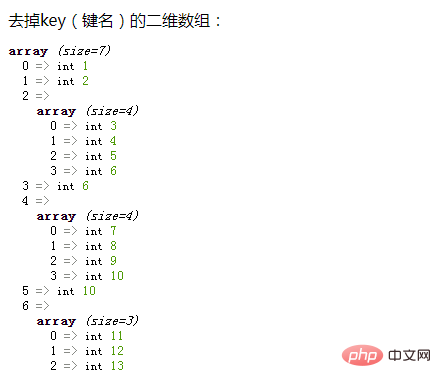# php中二维数组怎么去掉key（键名）`\$res=[];`

```foreach(\$arr as \$v){
//循环体代码
}```

• 如果是，则使用array_values()函数去掉内层数组的key（键名），并赋值给空数组\$res

• 如果不是，则直接将给外层元素的键值赋给给空数组\$res

```if(is_array(\$v)){
\$res[]=array_values(\$v);
}else{
\$res[]=\$v;
}```

```<?php
\$arr = array(
"a"=>1,
"b"=>2,
"c"=>array("c1"=>3,"c2"=>4,"c3"=>5,"c4"=>6),
"d"=>6,
"e"=>array("e1"=>7,"e2"=>8,"e3"=>9,"e4"=>10),
"f"=>10,
"g"=>array("g1"=>11,"g2"=>12,"g3"=>13)
);
echo "原二维数组：";
var_dump(\$arr);

\$res=[];
foreach(\$arr as \$v){
if(is_array(\$v)){
\$res[]=array_values(\$v);
}else{
\$res[]=\$v;
}
}
echo "去掉key（键名）的二维数组：";
var_dump(\$res);
?>```• 相关标签：php数组 php
• 相关文章

相关视频